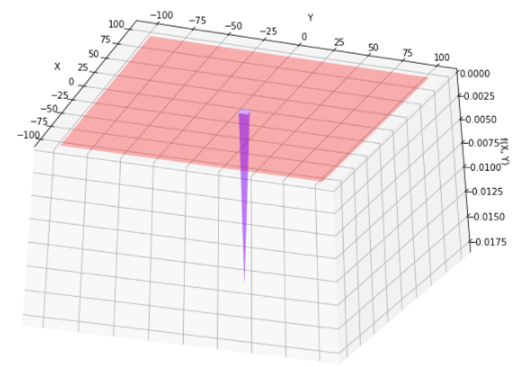top of page
Search

# EASOM FUNCTION

Updated: Jul 18, 2021Mathematical DefinitionInput Domain

The function is usually evaluated on xi ∈ [-100, 100], for all i = 1, 2.

Global Minima

The function has one global minimum f (x*) = =-1 at x*=(π, π)

Description and Features

• The function is continuous.

• The function is non scalable.

• The function is defined on 2-dimensional space.

• The function is unimodal.

• The function is differentiable.

• The function is non-separable.

• The Easom function has several local minima and the global minimum has a small area relative to the search space.

Python Implementation

```% Please forward any comments or bug reports in chat
Copyright 2021. INDUSMIC PRIVATE LIMITED.THERE IS NO WARRANTY, EXPRESS OR IMPLIED. WE DO NOT ASSUME ANY LIABILITY FOR THE USE OF THIS PROGRAM. If software is modified to produce derivative works, such modified software should be clearly marked. Additionally, user can redistribute it and/or modify it under the terms of the GNU General Public License. This program is distributed in the hope that it will be useful, but WITHOUT ANY WARRANTY. See the GNU General Public License for more details.
% for any support connect with us on help.indusmic@gmail.com
% Author: Ayushi Manish Shukla

from mpl_toolkits import mplot3d
import numpy as np
import matplotlib.pyplot as plt
import pandas as pd
import sympy as sy
import math
from matplotlib import cm
#def f(x1, x2): return -((np.cos(x1)*np.cos(x2))*np.exp(-(x1-math.pi)**2-(x2-math.pi)**2)
def f(x1, x2): return -(np.cos(x1)*np.cos(x2))*np.exp(-(x1-math.pi)**2-(x2-math.pi)**2)
x1 = np.linspace(-100,100)
x2 = np.linspace(-100,100)
X1, X2 = np.meshgrid(x1, x2)
plt.contour(X1, X2, f(X1,X2))
def plotter(E, A):
fig = plt.figure(figsize=[12,8])
ax = plt.axes(projection='3d')
ax.plot_surface(X1, X2, f(X1, X2), cmap='rainbow', alpha=0.3)
#ax.plot_wireframe(X1,X2,f(X1,X2),rcount=15,ccount=15)
ax.view_init(elev=E, azim=A)
ax.set_xlabel('X')
ax.set_ylabel('Y')
ax.set_zlabel('f(X, Y)')
print("solution 5")
plotter(45,45)
from ipywidgets import interactive
iplot = interactive(plotter, E = (-90, 90, 5),A = (-90, 90, 5))
iplot```

References:

 Jamil, Momin, and Xin-She Yang. "A literature survey of benchmark functions for global optimization problems." International Journal of Mathematical Modelling and Numerical Optimization 4.2 (2013): 150-194.